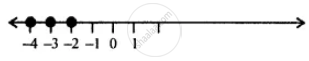# Solve and Graph the Solution Set on a Number Line: 8x – 8 < – 24 ; X ∈ Z - Mathematics

Sum

Solve and graph the solution set on a number line: 8x – 8 ≤ – 24 ; x ∈ Z

#### Solution

8x – 8 ≤ – 24

⇒ 8x - 8 + 8 ≤ - 24 + 8  ..(Adding 8 to both sides)

⇒ 8x ≤ -16

⇒ "8x"/8 <= (-16)/8   ..(Dividing both sides by 8)

⇒ x ≤ -2

∴ The required graph is:Is there an error in this question or solution?

#### APPEARS IN

Selina Concise Mathematics Class 8 ICSE
Chapter 15 Linear Inequations
Exercise 15 (B) | Q 5 | Page 175